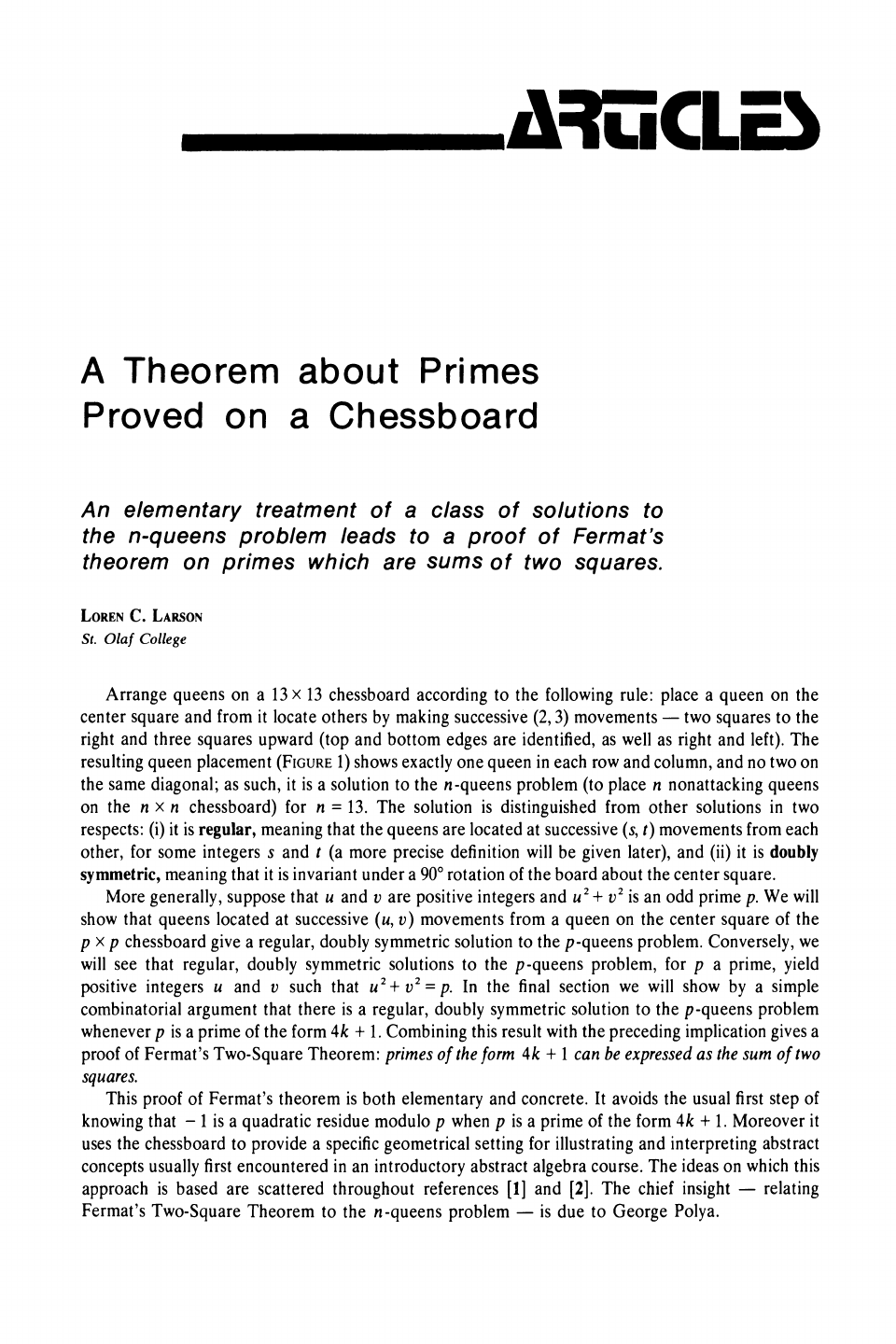The **n-queens problem** is a generalization of the 8-queens prob...
Fermat's theorem on sums of two squares says that an odd prime $p$ ...
The set of all integers which have the same remainder when divided ...
the only symmetrical solution!
This result is not obvious, particularly for someone without any kn...
Since we are going to place n queens in an nxn board, every queen n...
For the example in Figure 1, since $n=13$ if we choose $r=2$, $(13,... Note that$(n,c) + \langle (1,d) \rangle $generates positions of t... As seen before two numbers a and b are relatively prime if and only...$(p,c)+ \langle(1, d)\rangle = (p + i, c+ id)$where$i=1,2,...,n$.... If$d=p$, d is obviously not relatively prime to p. If$d=p-1\$, ...A
Theorem
Proved on
a Chessboard
An
elementary treatment
of
a
class of solutions to
the
proof of Fermat's
theorem on
primes
which
are
sums
of
two squares.
LOREN C. LARSON
St.
Olaf College
Arrange queens
on a 13
x
13
chessboard according to the following rule: place a queen on the
center
square
and from it
locate others
by making successive (2, 3) movements
-
two squares to the
right
and
three
squares
upward (top and bottom edges are identified, as well as right and left). The
resulting queen placement
(FIGURE 1) shows exactly one queen
in
each row and column, and no two on
the
same
diagonal;
as
such,
it
is
a
solution
to the
n-queens problem (to place n nonattacking queens
on
the
n
x
n
chessboard)
for
n
=
13. The
solution
is
distinguished
from other solutions
in
two
respects: (i)
it is
regular,
meaning
that the
queens
are
located at successive
(s, t)
movements
from each
other,
for
some
integers
s and
t
(a
more
precise
definition
will
be
given later),
and
(ii)
it
is
doubly
symmetric, meaning
that it is invariant under a 900
rotation of
the
board
square.
More
generally, suppose
that u and
v
are
positive integers
and
u2+ v2 is
an odd
prime p.
We will
show
that
queens
located at
successive
(u, v)
movements from
a
queen
on the center
square
of
the
p
x
p chessboard give a
regular, doubly symmetric solution to the p-queens problem. Conversely, we
will see
that regular,
doubly symmetric
solutions
to
the
p-queens problem,
for
p
a
prime, yield
positive integers
u
and v such that
u2+ v2
=p.
In the
final section
we will show
by
a
simple
combinatorial
argument
that there
is
a
regular, doubly symmetric
solution to the
p-queens problem
whenever p is a prime of the
form
4k
+
1. Combining
this result with
the
preceding implication gives
a
proof
of Fermat's
Two-Square
Theorem:
primes of
the
form
4k
+
1 can be
expressed
as the sum
of
two
squares.
This
proof
of
Fermat's theorem
is
both
elementary
and concrete.
It
avoids the
usual first
step
of
knowing
that
-
1 is
a
residue modulo
p
when
p
is
a
prime
of
the
form
4k
+
1.
Moreover
it
uses
the
chessboard to
provide
a
specific geometrical setting
for
illustrating
and
interpreting
abstract
concepts usually
first
encountered
in
an
introductory
abstract
algebra
course. The ideas on
which this
approach
is
based are
scattered
throughout
references

and
.
The
chief
insight
-
relating
Fermat's
Two-Square
Theorem to the n-queens problem
-
is due to George Polya.
This content downloaded from 129.128.216.34 on Wed, 13 Nov 2013 15:26:47 PM
All use subject to JSTOR Terms and Conditions